### 4. CROSS-CORRELATION ANALYSIS

Cross-correlation analysis is the tool most commonly used in the analysis of multiple time series. Because its application to astronomical time series is often misunderstood and has historically been rather contentious, it merits special attention. Important steps in the development of cross-correlation analysis as applied to AGN variability studies can be found in the literature 27, 28, 20, 49, 39 .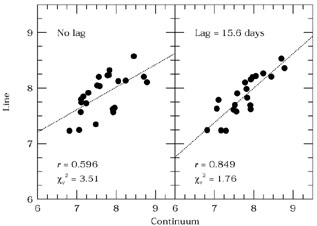Figure 22. The left-hand panel shows simultaneous measurements of H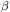emission-line and optical continuum fluxes for the Seyfert galaxy Mrk 335 69. These consist of 24 measurements made on an approximately weekly basis over one observing season. The right-hand panel shows the same emission-line fluxes paired with continuum values from 15.6 days earlier; i.e., the emission-line fluxes are better correlated with earlier rather than current continuum values on account of light travel-time delays. When the time lag is taken into account, the fit improves.(31)

where there are N pairs of values (xi, yi) and their respective means areand. When the two variables x and y are perfectly correlated, r = 1. If they are perfectly anticorrelated, r = -1. If they are completely uncorrelated, r = 0. For the data shown in the left panel of Fig. 22, r = 0.596; for 24 pairs of points, as shown here, this means that the correlation is significant at the99.8% confidence level (i.e., the chance that the two variables are in fact completely uncorrelated and the correlation we find is spurious is less than 0.02%. Confidence levels for linear correlation can be found in standard statistical tables 6).

While this is quite a good correlation, we see something more remarkable if we plot both variables as functions of time (i.e., as light curves), as seen in Fig. 23. We see that the patterns of variation are very similar, except that the emission-line light curve is delayed in time, or "lagged," relative to the continuum light curve. It is obvious that the correlation between the continuum and emission-line fluxes would be even better if we allowed a linear shift in time between the two light curves in order to line up their prominent maxima and minima. This is what cross-correlation does.Figure 23. The Hemission-line and optical continuum fluxes for Mrk 335, as shown in Fig. 22, are plotted as a function of time. It is clear from the figure that the continuum and emission-line fluxes are well-correlated, and that the correlation can be improved by a linear shift in time of one time series relative to the other. The optimum linear correlation occurs by shifting the emission-line light curve backwards by 15.6 days.

The first operational problem in computing a cross-correlation is also immediately apparent: since each point in one light curve must be paired with a point in the other light curve, it is obvious that the data should be regularly spaced. The cross-correlation is then evaluated as a function of the spacing between the interval between data pointst using the pairs [x(ti), y(ti + Nt)] for all integers N. Unfortunately, regularly sampled data are almost never found in Astronomy; ground-based programs have weather to contend with, and even satellite-based observations are almost never regularly spaced in time. The essence of the cross-correlation problem in Astronomy is dealing with time series that are not evenly sampled. Moreover, the light curves are often limited in extent and are noisy.

For well-sampled series as in Fig. 23, the sampling problem can be dealt with in a straightforward fashion. The simple, effective solution is to interpolate one series between the actual data points, and use the interpolated points in the cross-correlation. We illustrate this schematically in Fig. 24. We can then compute the cross-correlation function CCF(), as shown in Fig. 25, and the step size we use foris now somewhat arbitrary. At each value of the lagwe compute r as in Eq. (31). For the example we have been using, we find that the CCF is maximized when points in the continuum light curve are matched to those in the emission-line light curve with a delay of 15.6 days. If we plot the shifted emission-line values versus the continuum values (as we have done in the right-hand panel of Fig. 22) and again perform a linear correlation analysis, we find that the fit has improved, with r = 0.849 and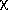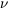2 = 1.76.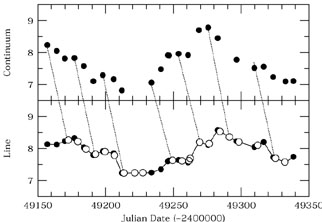Figure 24. Continuum and emission-line light curves for Mrk 335, as in Fig. 23. This illustrates the interpolation method commonly used in cross-correlation. In this figure, the emission-line light curve is made continuous through linear interpolation between data points. Actual continuum observations are then paired with interpolated emission-line values to compute the correlation coefficient for a particular time delay. In this example, we show interpolated emission-line fluxes that are time-delayed relative to the continuum by 15.6 days, which is the value at which the cross-correlation function peaks. As a visual aid, dotted lines join a few of the data pairs. Notice how the first few points of the emission-line series and the last few points of the continuum series remain unused.Figure 25. The interpolation cross-correlation function for the Mrk 335 data shown in the previous figures. The shaded area indicates points with values r0.8rpeak, which are the points used in computing the centroid, which is also indicated.